Next: Adattamento di curve ai Up: Matrice di covarianza di Previous: Normalization uncertainty   Indice

### General case

Let us assume there are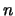independently measured values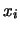and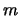calibration constants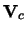with their covariance matrix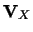. The latter can also be theoretical parameters influencing the data, and moreover they may be correlated, as usually happens if, for example, they are parameters of a calibration fit. We can then include thein the vector that contains the measurements andin the covariance matrix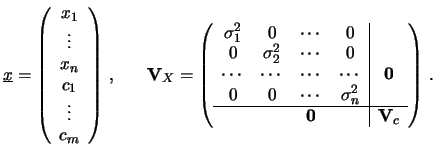: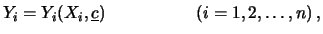(14.33)

The corrected quantities are obtained from the most general function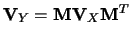(14.34)

and the covariance matrix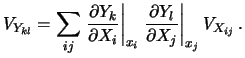from the covariance propagation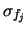.

As a frequently encountered example, we can think of several normalization constants, each affecting a subsample of the data - as is the case where each of several detectors measures a set of physical quantities. Let us consider just three quantities (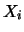) and three uncorrelated normalization standard uncertainties (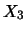), the first common to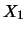and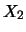, the second toand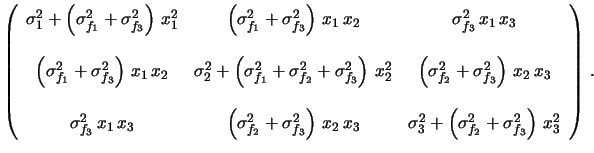and the third to all three. We get the following covariance matrix: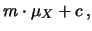(14.35)Next: Adattamento di curve ai Up: Matrice di covarianza di Previous: Normalization uncertainty   Indice
Giulio D'Agostini 2001-04-02